# StoichiometryPage 2

#### WATCH ALL SLIDES

Use mole ratio

Get the answer in moles and then

Convert to Mass. [Simple Arithetic]

Hello!

If you are given a mass in the problem, you will need to convert this to moles first. Ok?

Slide 12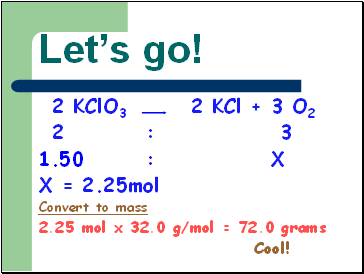Let’s go!

2 KClO3 2 KCl + 3 O2

2 : 3

1.50 : X

X = 2.25mol

Convert to mass

2.25 mol x 32.0 g/mol = 72.0 grams

Cool!

Slide 13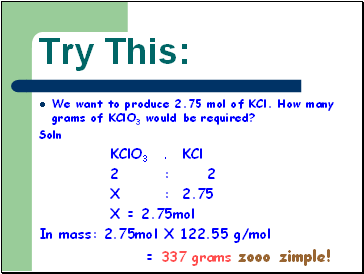Try This:

We want to produce 2.75 mol of KCl. How many grams of KClO3 would be required?

Soln

KClO3 : KCl

2 : 2

X : 2.75

X = 2.75mol

In mass: 2.75mol X 122.55 g/mol

= 337 grams zooo zimple!

Slide 14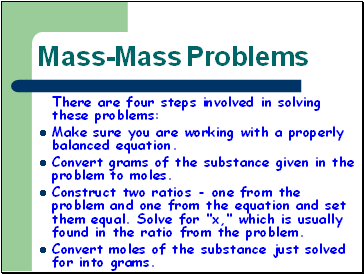## Mass-Mass Problems

There are four steps involved in solving these problems:

Make sure you are working with a properly balanced equation.

Convert grams of the substance given in the problem to moles.

Construct two ratios - one from the problem and one from the equation and set them equal. Solve for "x," which is usually found in the ratio from the problem.

Convert moles of the substance just solved for into grams.

Slide 15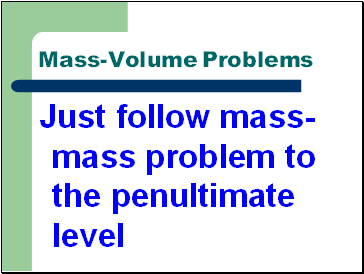## Mass-Volume Problems

Just follow mass-mass problem to the penultimate level

Slide 16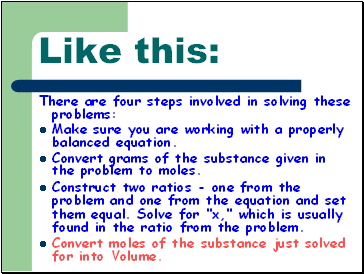Like this:

There are four steps involved in solving these problems:

Make sure you are working with a properly balanced equation.

Convert grams of the substance given in the problem to moles.

Construct two ratios - one from the problem and one from the equation and set them equal. Solve for "x," which is usually found in the ratio from the problem.

Convert moles of the substance just solved for into Volume.

Slide 17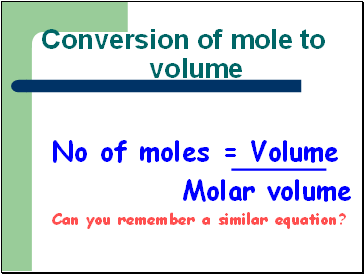## Conversion of mole to volume

No of moles = Volume

Molar volume

Can you remember a similar equation?

Slide 18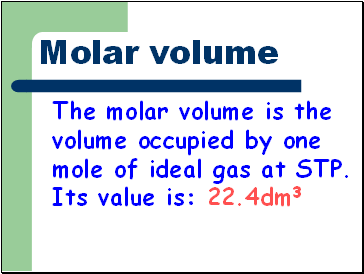## Molar volume

The molar volume is the volume occupied by one mole of ideal gas at STP. Its value is: 22.4dm3

Slide 19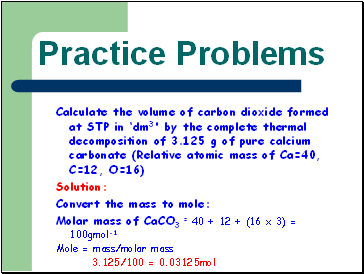## Practice Problems

Calculate the volume of carbon dioxide formed at STP in ‘dm3' by the complete thermal decomposition of 3.125 g of pure calcium carbonate (Relative atomic mass of Ca=40, C=12, O=16)

Solution:

Convert the mass to mole:

Molar mass of CaCO3 = 40 + 12 + (16 x 3) = 100gmol-1

Mole = mass/molar mass

Go to page:
1  2  3